Parents and Teachers: Support Ducksters by following us onor.

Kids Math

Circle Geometry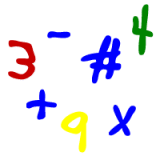Skills needed:
• Multiplication
• Basic Geometry
A circle is an important shape in geometry. There are circles all around us in the real world. We can learn a lot about the real world and how it works by understanding circles.

First, what is the official definition of a circle?

A circle is a shape that is made up of all the points on a plane (a flat surface) that are the same distance from a given point.

What does that mean?

Okay, so let's start out with the "given point". Let's pick a point on a flat surface, like this screen, for instance. We'll call it point P.

Now pick all the other points on the screen that are the exact same distance R away from point P.

Watch as we add in equal distanced points in the movie below: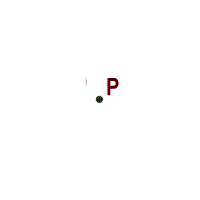Wow, we get a circle!

Terms used in circle geometry
 Radius - The radius is the distance from the center point to the edge of the circle. This is the same distance R we used to make the circle in our definition.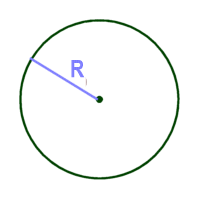Diameter - The diameter is a straight line that goes across the circle and through the center. It's twice the length of the radius. This gives you our first math equation for the circle: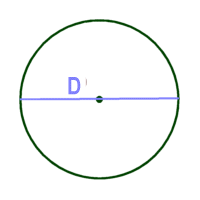D = 2 x R

Also,

R = D/2

So the diameter is two times the radius and the radius is one half of the diameter.
 Circumference - The circumference is the distance around the circle. We use the following formula to figure the circumference: c = d * π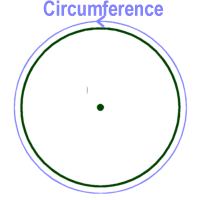Uh oh! What is this symbol π???

This symbol is called pi. It stands for a number that we use with circles. We won't go into the details right now, but let's just agree to use it and that it works for now. Okay?

π = 3.14…..

The decimals actually go on for a long time (forever), but we will round it off to 3.14. It's close enough.

Back to circumference; There are a few handy formulas we will use:

c = d * π
c = 2 * π * r

Where c = circumference, d = diameter, and r = radius.

Other Circle Geometry terms
 Sector - A sector is a section of the circle made by two different radii. Sort of like a piece of pizza or pie. Chord - A chord is a line segment that joins two points on the circle. The diameter is a special type of chord that goes through the center point of the circle.More Geometry Subjects

Circle
Polygons
Triangles
Pythagorean Theorem
Perimeter
Slope
Surface Area
Volume of a Box or Cube
Volume and Surface Area of a Sphere
Volume and Surface Area of a Cylinder
Volume and Surface Area of a Cone
Angles glossary
Figures and Shapes glossary

Back to Kids Math

Back to Kids StudyFollow us onor